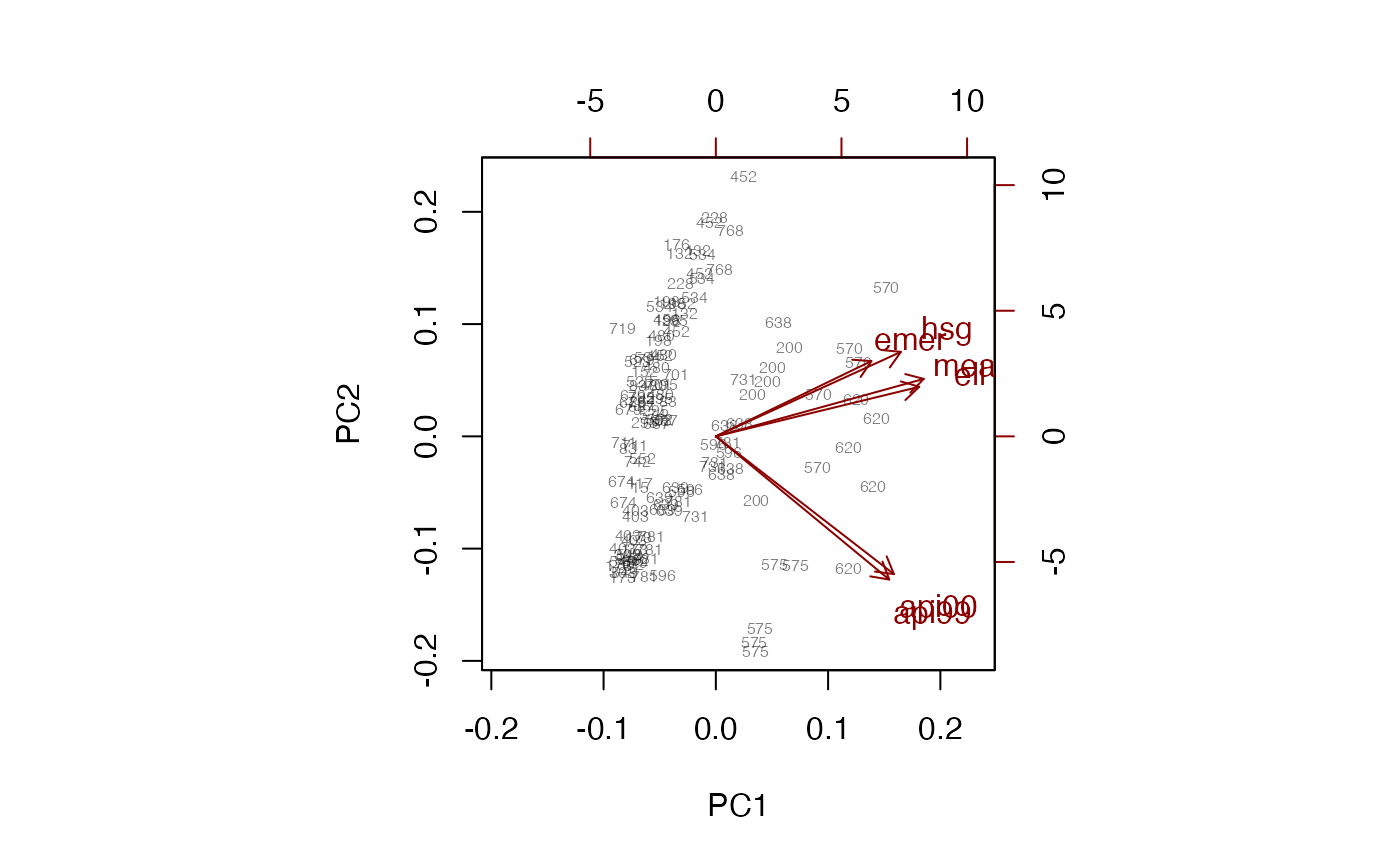Computes principal components using the sampling weights.

svyprcomp(formula, design, center = TRUE, scale. = FALSE, tol = NULL, scores = FALSE, ...)
# S3 method for svyprcomp
biplot(x, cols=c("black","darkred"),xlabs=NULL,
weight=c("transparent","scaled","none"),
max.alpha=0.5,max.cex=0.5,xlim=NULL,ylim=NULL,pc.biplot=FALSE,
expand=1,xlab=NULL,ylab=NULL, arrow.len=0.1, ...)

## Arguments

formula

model formula describing variables to be used

design

survey design object.

center

Center data before analysis?

scale.

Scale to unit variance before analysis?

tol

Tolerance for omitting components from the results; a proportion of the standard deviation of the first component. The default is to keep all components.

scores

Return scores on each component? These are needed for biplot.

x

A svyprcomp object

cols

Base colors for observations and variables respectively

xlabs

Formula, or character vector, giving labels for each observation

weight

How to display the sampling weights: "scaled" changes the size of the point label, "transparent" uses opacity proportional to sampling weight, "none" changes neither.

max.alpha

Opacity for the largest sampling weight, or for all points if weight!="transparent"

max.cex

Character size (as a multiple of par("cex")) for the largest sampling weight, or for all points if weight!="scaled"

xlim,ylim,xlab,ylab

Graphical parameters

expand,arrow.len

See biplot

pc.biplot

See link{biplot.prcomp}

...

Other arguments to prcomp, or graphical parameters for biplot

## Value

svyprcomp returns an object of class svyprcomp, similar to class prcomp but including design information

prcomp, biplot.prcomp

## Examples

data(api)
dclus2<-svydesign(id=~dnum+snum, fpc=~fpc1+fpc2, data=apiclus2)

pc <- svyprcomp(~api99+api00+ell+hsg+meals+emer, design=dclus2,scale=TRUE,scores=TRUE)
pc
#> Standard deviations (1, .., p=6):
#>  2.00614662 1.06784643 0.72819673 0.51593850 0.18862407 0.05511512
#>
#> Rotation (n x k) = (6 x 6):
#>             PC1        PC2         PC3         PC4          PC5         PC6
#> api99 0.3831145 -0.5943589  0.08306768 -0.06979896  0.022302291 -0.69834894
#> api00 0.3934925 -0.5718779  0.03836692 -0.07704907 -0.008208835  0.71459312
#> ell   0.4493746  0.2056601 -0.25640658  0.58763687  0.587114958  0.00100877
#> hsg   0.4089487  0.3506756 -0.21317497 -0.77628465  0.248055264 -0.01395416
#> meals 0.4594419  0.2384659 -0.32112577  0.19296434 -0.768508662 -0.03293318
#> emer  0.3436909  0.3123423  0.88165644  0.06341416 -0.050933992  0.01962417
biplot(pc, xlabs=~dnum, weight="none")biplot(pc, xlabs=~dnum,max.alpha=1)biplot(pc, weight="scaled",max.cex=1.5, xlabs=~dnum)